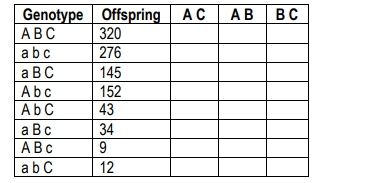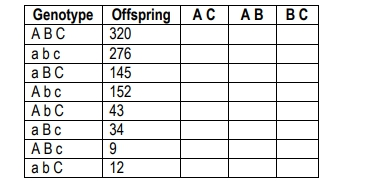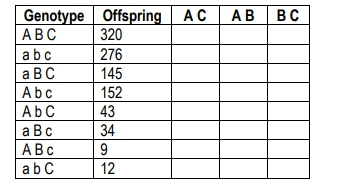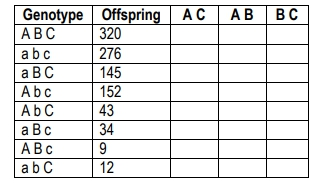Start typing, then use the up and down arrows to select an option from the list.# Genetics

Learn the toughest concepts covered in biology with step-by-step video tutorials and practice problems by world-class tutors

1

#### concept

Trihybrid Cross26m
Play a video:
2
Problem

The following table shows data from a cross (ABC x abc) examining three genes (a, b, and c). Calculate the recombination frequency for A and B3
Problem

The following table shows data from a cross examining three genes (a, b, and c). Calculate the recombination frequency for A and C4
Problem

The following table shows data from a cross examining three genes (a, b, and c). Calculate the recombination frequency for B and C5
Problem

The following table shows data from a cross examining three genes (a, b, and c). Determine the order of genes